# Analysis of earthquake distribution in the past 10 years with Python~

2022-02-01 03:03:51

Hello everyone , I'm brother CAI .

Some time ago, something happened in the Yellow Sea `5.2 level ` The earthquake , At that time, friends in East China were all “ shock ” 了 .today , Let's take a look at the past `10` Distribution of earthquakes in ！

## 1. Data acquisition

Directly in ` China earthquake networks ` You can download it to In the past 10 year The earthquake data .

China earthquake networks ：

news.ceic.ac.cn/index.html?…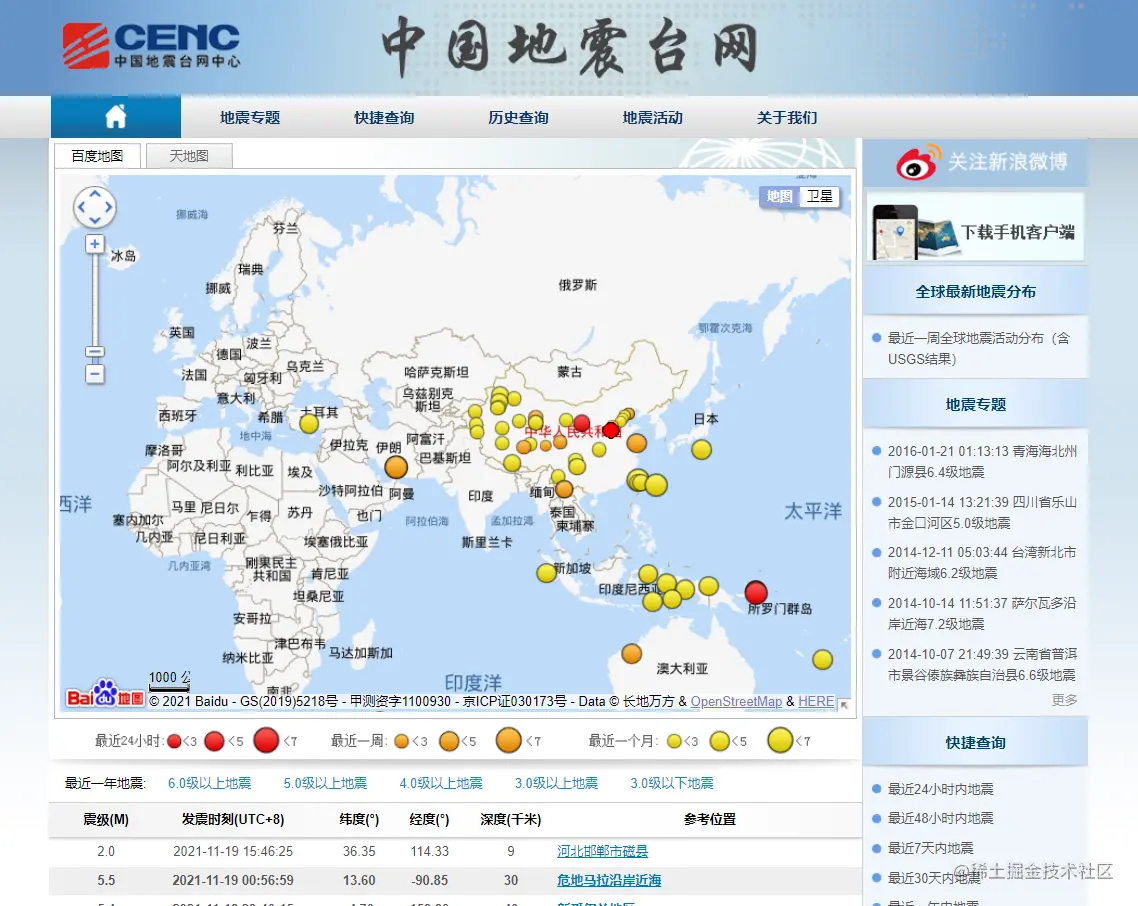You're right here History query Interior selection Time range After the inquiry , Click on Save to local You can get the data .

There are local data previews as follows ：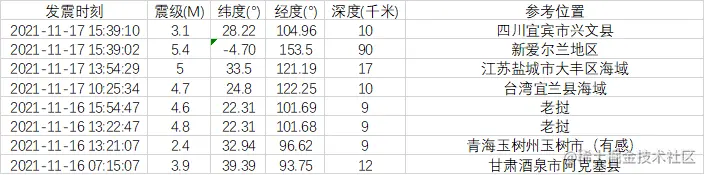## 2. Data processing

In data acquisition , We found that the data obtained contains Time Magnitude Longitude and latitude depth as well as Reference position . The longitude and latitude are under Baidu map , Considering that the longitude and latitude of different maps will be different , And our subsequent drawing adopts Gaode map , So here we need to convert the latitude and longitude .

### 2.1. Latitude and longitude conversion

Gaode open platform has corresponding Latitude and longitude conversion Of `API`, We can call （ The free quota is enough ）.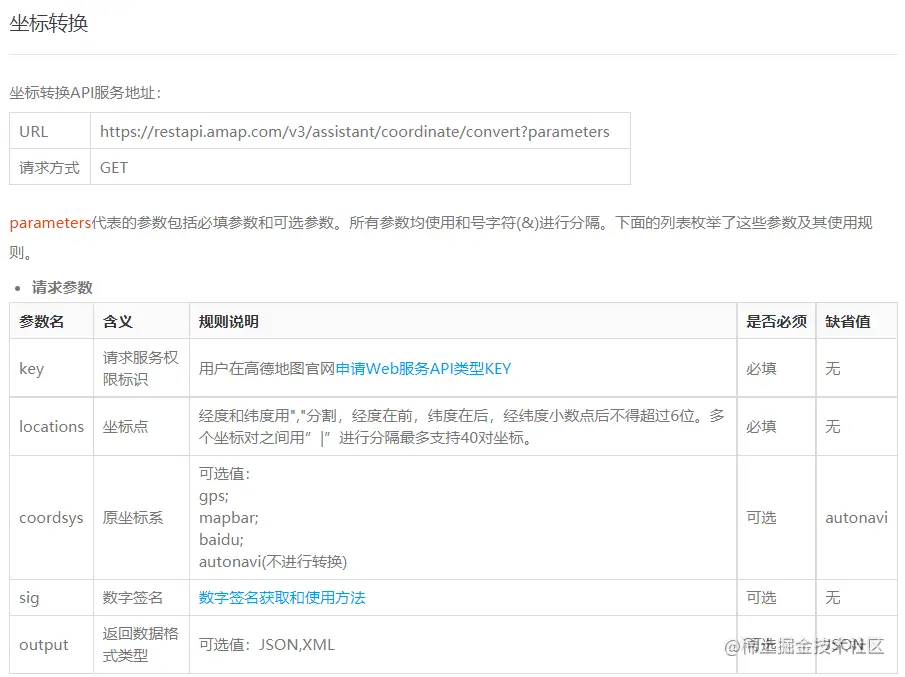``````import requests
import pandas as pd

longitude_list = []
latitude_list = []
#  Baidu map longitude and latitude is converted to Gaode map longitude and latitude
for i , location in enumerate(df[[' longitude (°)',' latitude (°)']].values):
location = str(location)+','+str(location)
url = 'https://restapi.amap.com/v3/assistant/coordinate/convert?'

parames = {
'locations':location,
'coordsys':'baidu',
'key':' Yours key',
}

r = eval(requests.get(url, params=parames).json()['locations'])
#  longitude
longitude_list.append(r)
#  latitude
latitude_list.append(r)
print(f'\r{i+1}',end='')

df[' longitude (°)'] = longitude_list
df[' latitude (°)'] = latitude_list
Copy code ``````

such , We have successfully converted the longitude and latitude coordinates of Baidu map system into the longitude and latitude coordinates of Gaode map system .

### 2.2. Data processing at the time of earthquake occurrence

For the moment of earthquake , I want to be accurate to the month and hour , For subsequent statistical analysis .

``````#  Convert to time format
df[' The moment of the earthquake '] = pd.to_datetime(df[' The moment of the earthquake '])
#  Get the date
df[' month '] = df[' The moment of the earthquake '].apply(lambda x: str(x)[:7])
#  For hours
df[' Hours '] = df[' The moment of the earthquake '].dt.hour
Copy code ``````

Here, I feel that my operation of getting the month and year is a little `low`, If you have a better plan, please leave a message , I want to learn .

### 2.3. Earthquake location （ Provinces ）

Because the reference position in the original data can not be easily analyzed to the province and city , I'm going to use the longitude and latitude information through Gaode's `API` To get . Refer to previous 《》.

``````citys = []
provinces = []
for i , location in enumerate(df[[' longitude (°)',' latitude (°)']].values):
location = str(location)+','+str(location)
url = 'https://restapi.amap.com/v3/geocode/regeo?'
params = {
'location':location,
'key':' Yours key',
'extensions':'base',
'batch':'false',
}

r = requests.get(url, params=params)
data = r.json()['regeocode']
if len(city)==0:
city = province
citys.append(city)
provinces.append(province)
print(f'\r{i+1}',end='')
df[' City '] = citys
df[' province '] = provinces
Copy code ``````

After the above treatment , We finally got the following data ：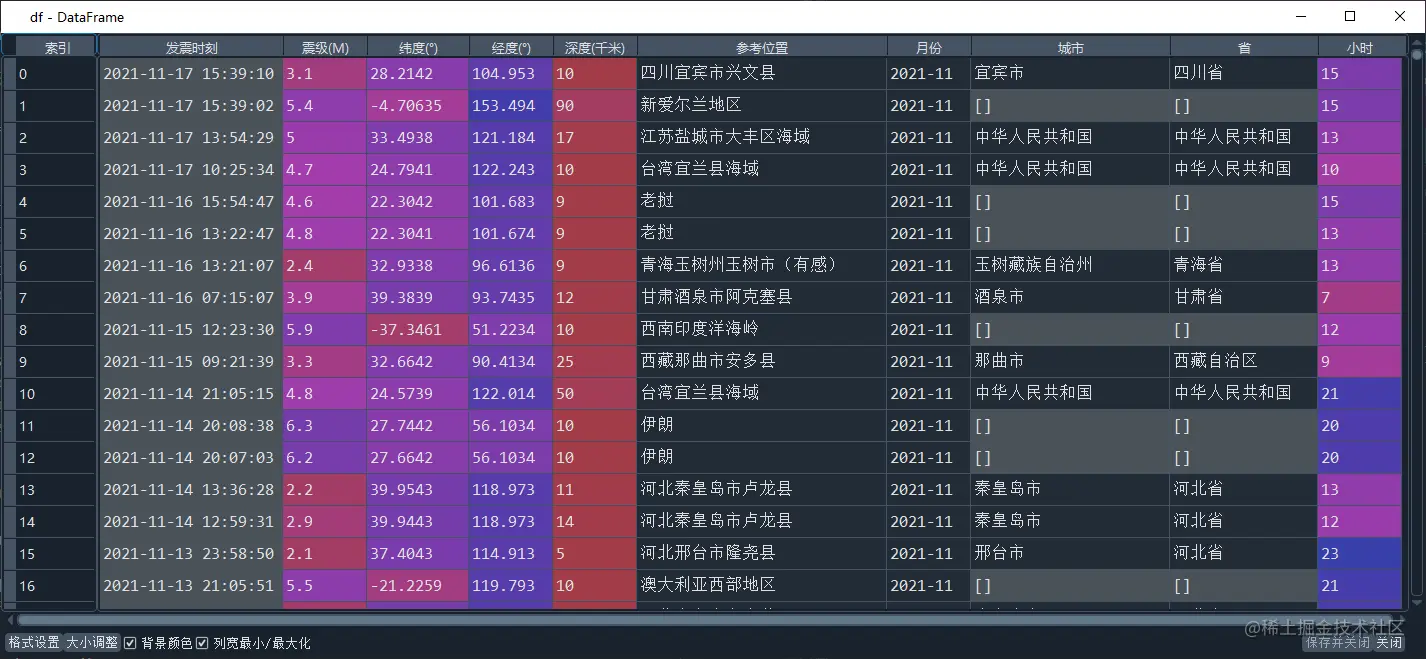## 3. Statistics and visualization

In this part, we only make a simple statistical display , Do not make similar predictions or other in-depth analysis , After all, based on the existing raw data, we can't draw much valuable conclusions .

### 3.1. Number of earthquakes over the years

Number of small and medium earthquakes

according to `[2, 4.6]` As the range of medium and small earthquakes , near 10 In all 6188 Time , Average annual 600 Remaining times ！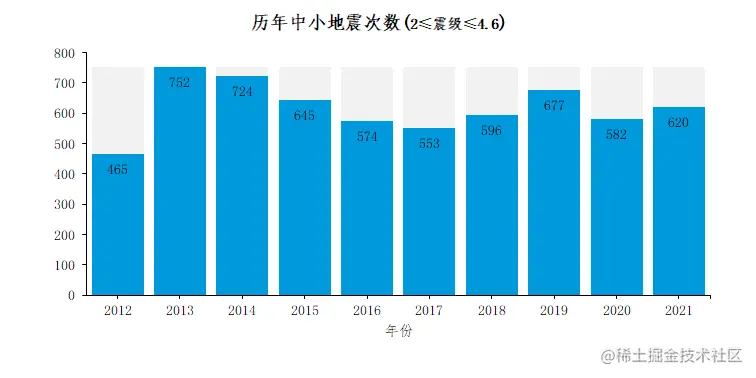``````#  Small and medium earthquakes （[2,4.6]）
df[' year '] = df[' The moment of the earthquake '].dt.year
df_cn = df[df[' province ']!='[]']
df_xiao = df_cn.query('2<=` Magnitude (M)`<=4.6')
df_xiao.groupby(' year ')[' The moment of the earthquake '].count().to_frame(' frequency ').reset_index()
Copy code ``````

Number of destructive earthquakes

according to `[4.7, ∞]` As the range of medium and small earthquakes , near 10 In all 505 Time , Average annual 50 Remaining times ！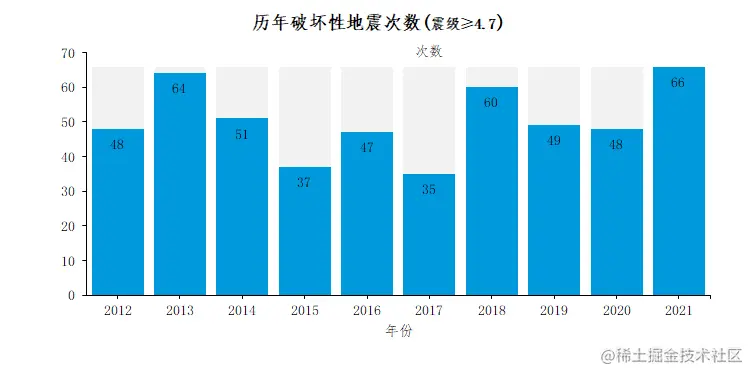### 3.2. Number of earthquakes in each province

Considering that some earthquakes occur in the sea , It is unified into Chinese waters , In addition, we don't participate in the events that take place abroad .

in addition , We only see Destructive earthquake Distribution , It can be found that in our country xinjiang Tibet yunnan Taiwan and sichuan It is a zone with high earthquake incidence ！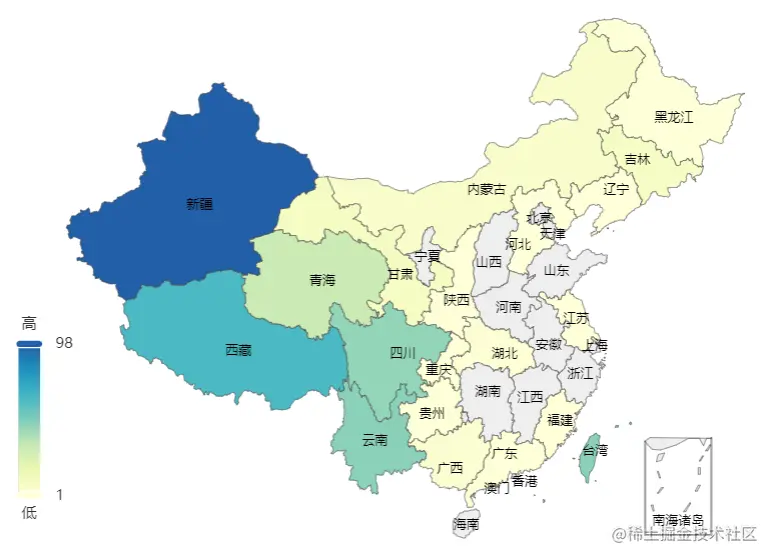``````#  The provinces   Destructive earthquake
df_province = df_cn.query('` Magnitude (M)`>=4.7').groupby(' province ')[' The moment of the earthquake '].count().to_frame(' frequency ').sort_values(by=' frequency ',ascending=False).reset_index()
Copy code ``````
Province Number of destructive earthquakes
xinjiang 98
Tibet 63
yunnan 47
Taiwan 47
sichuan 45
qinghai 32
gansu 10
Ji Lin 9
Inner Mongolia 7
guangxi 4
hubei 3
guangdong 2
hebei 2
guizhou 2
Chongqing 2
heilongjiang 2
jiangsu 1
fujian 1
liaoning 1
shaanxi 1

### 3.3. Number of earthquakes in each city

In our country `233` There have been earthquakes in three cities , Most of the cities with the most earthquakes are concentrated in xinjiang sichuan .

notes ： The statistics here are all seismic data

Like Xinjiang ` Kizilsu Kirgiz Autonomous Prefecture `` Hotan Area `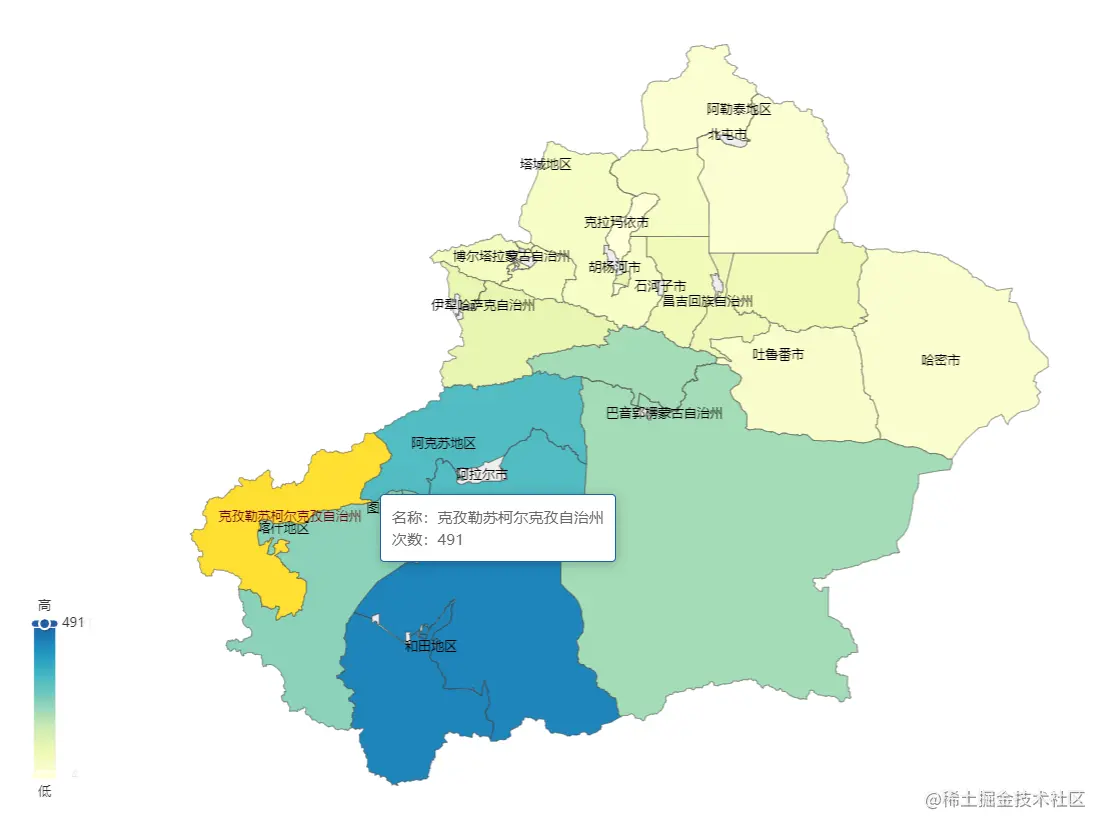Sichuan ` Yibin ` and ` Ya'an ` etc.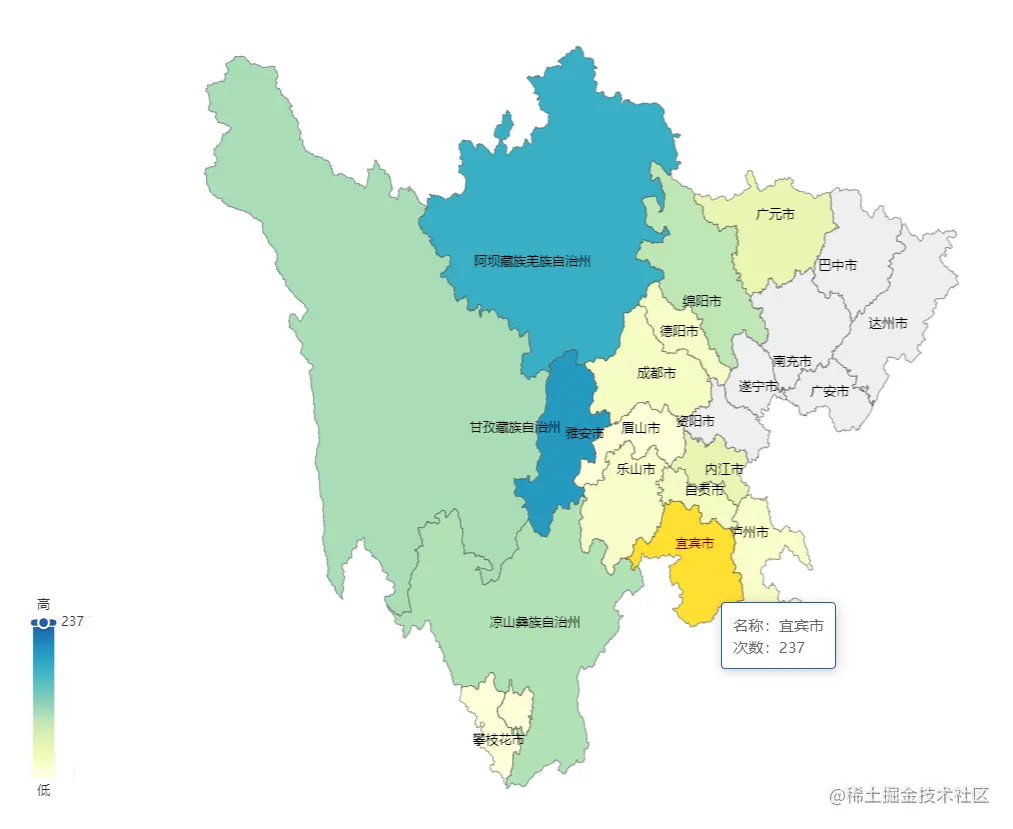province City frequency
Xinjiang Uygur Autonomous Region Kizilsu Kirgiz Autonomous Prefecture 491
Xinjiang Uygur Autonomous Region Hotan Area 431
Xinjiang Uygur Autonomous Region Aksu Area 308
Tibet Autonomous Region Naqu city 257
Sichuan Province Yibin City 237
Xinjiang Uygur Autonomous Region Kashgar area 234
Xinjiang Uygur Autonomous Region Bayingolin Mongol Autonomous Prefecture 206
Sichuan Province Ya'an City 188
Taiwan Province Taiwan Province 167
Sichuan Province Aba Tibetan and Qiang Autonomous Prefecture 167

【 Moving graph 】

### 3.4. Scatter diagram of earthquake distribution

We only see Destructive earthquake Distribution , Draw according to the longitude and latitude coordinate information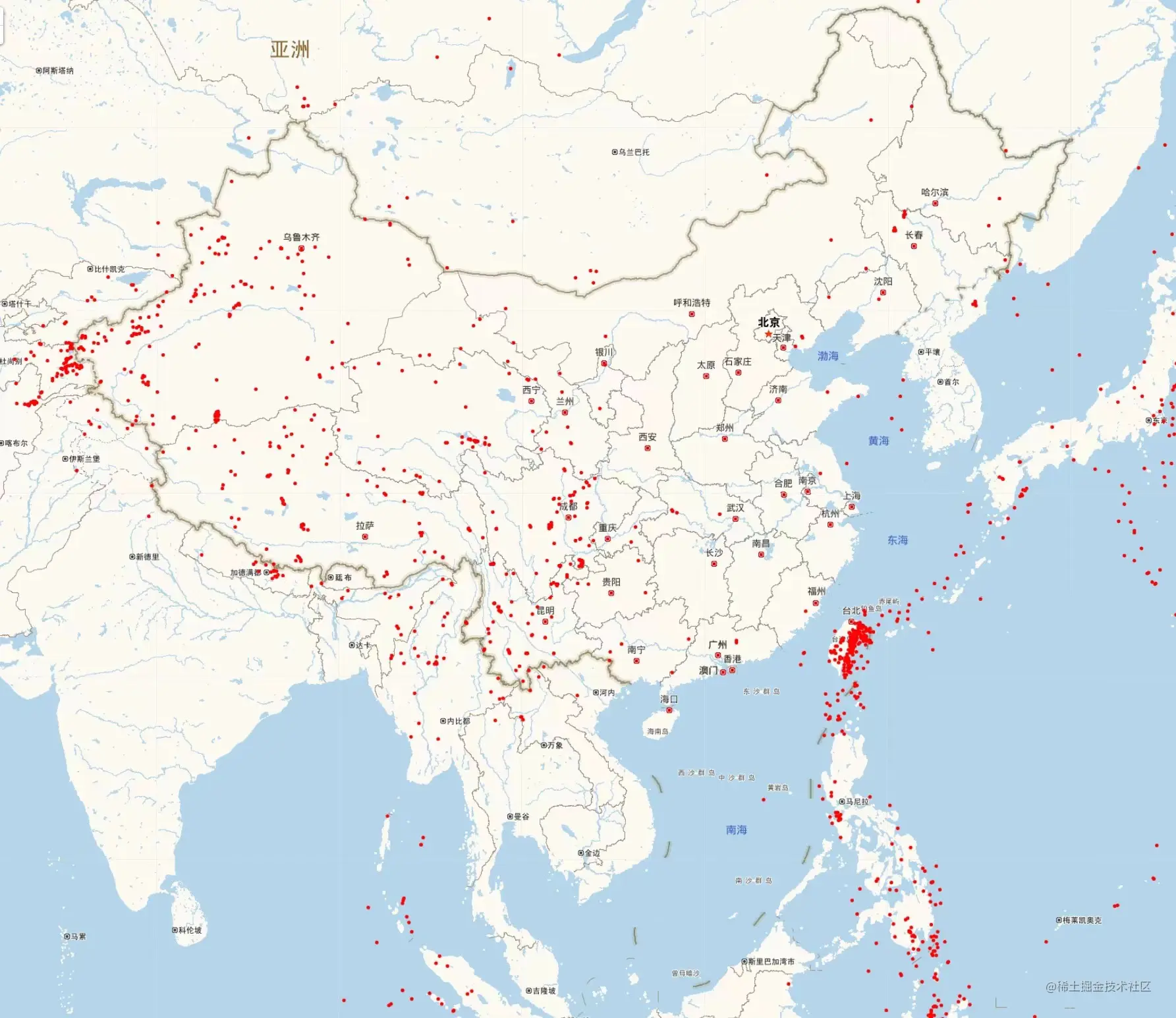``````import folium.plugins as plugins
import folium

df = df[df[' Magnitude (M)']>=4.7]
ss1 = [[latitude,longitude] for latitude,longitude in df[[' latitude (°)', ' longitude (°)']].values.tolist()]
m = folium.Map([39.904989, 116.405285],
tiles='https://webrd01.is.autonavi.com/appmaptile?&x={x}&y={y}&z={z}&lang=zh_cn&size=1&scale=1&style=7',
zoom_start=6,
attr=' Gao de ')
groups = folium.FeatureGroup('')
for l,label in zip(ss1,df[' Reference position '].to_list()):
folium.CircleMarker(
location=l,
color='red',
fill=True,
fill_color='red',
fill_opacity=0.4,
popup=folium.Popup(html=label,max_width=100),
)
)
m.save(' Earthquake distribution .html')
Copy code ``````

### 3.5. Address distribution diagram

You can see the southwest of Xinjiang , And the eastern waters of Taiwan Province are earthquake prone areas .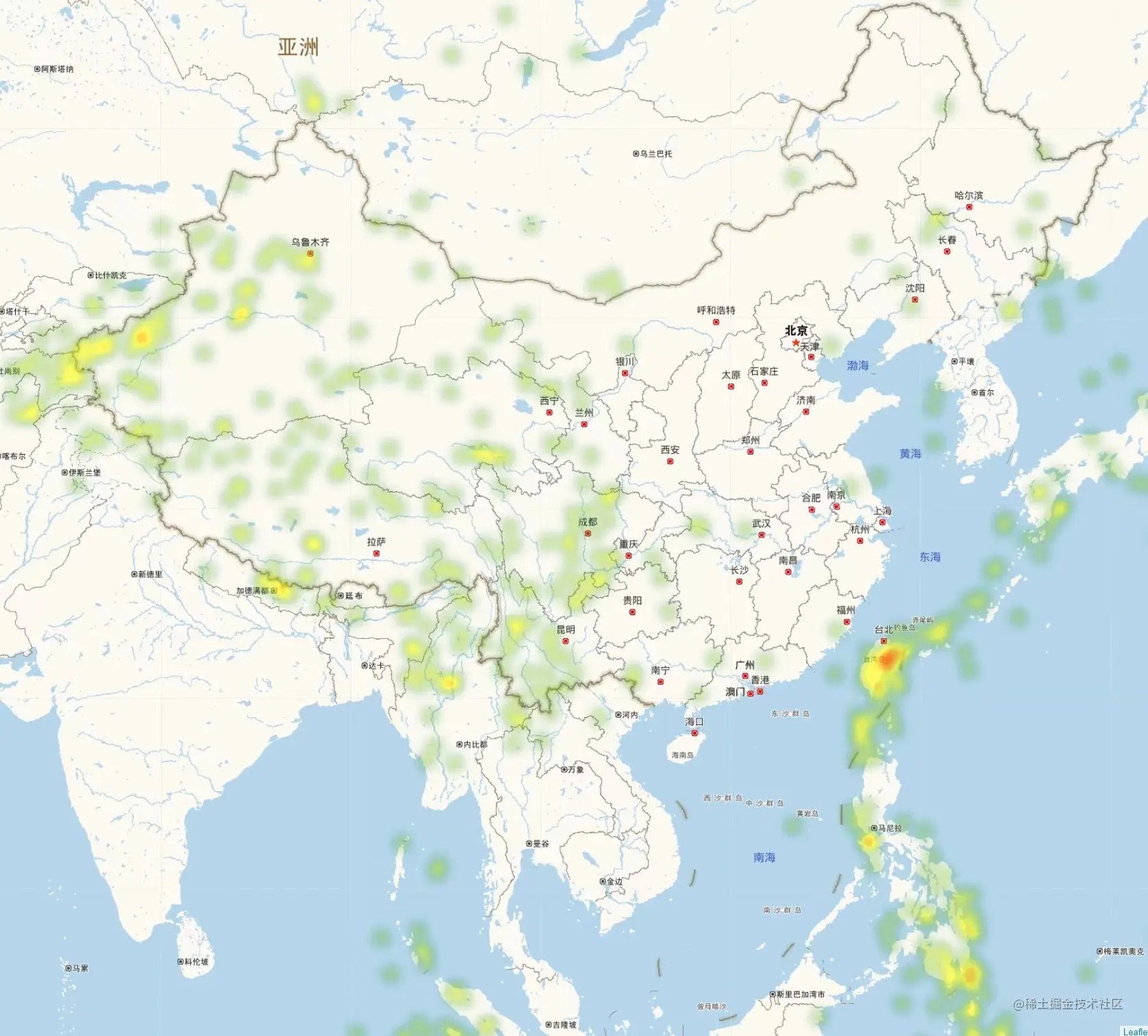``````data_all = df[[' latitude (°)', ' longitude (°)',' Magnitude (M)']].values.tolist()
m = folium.Map([39.904989, 116.405285],
tiles='https://webrd01.is.autonavi.com/appmaptile?&x={x}&y={y}&z={z}&lang=zh_cn&size=1&scale=1&style=7',
zoom_start=6,
attr=' Gao de ')
hm = plugins.HeatMap(data_all, radius=10,
gradient={.1: 'green', .6: 'yellow', 1: 'red'},
)
hm.save(' Seismic thermal map .html')
Copy code ``````

### 3.6. near 10 Annual monthly earthquake distribution map （ dynamic ）

notes ： The statistics here are all seismic data

【 Video Number 】

Draw code

``````data_move = []
date_list = df[' month '].sort_values().unique()
for month in date_list:
data_move.append(df[df[' month '] == month][[' latitude (°)', ' longitude (°)',' Magnitude (M)']].values.tolist())
m = folium.Map([39.904989, 116.405285],
tiles='https://webrd01.is.autonavi.com/appmaptile?&x={x}&y={y}&z={z}&lang=zh_cn&size=1&scale=1&style=7',
zoom_start=6,
attr=' Gao de ')
time_index = df[' month '].sort_values().unique().tolist()
hm = plugins.HeatMapWithTime(data_move,
index=time_index,

hm.save(' Seismic dynamic thermodynamic diagram .html')
Copy code ``````

## 4. Supplementary knowledge

Distribution map of seismic zone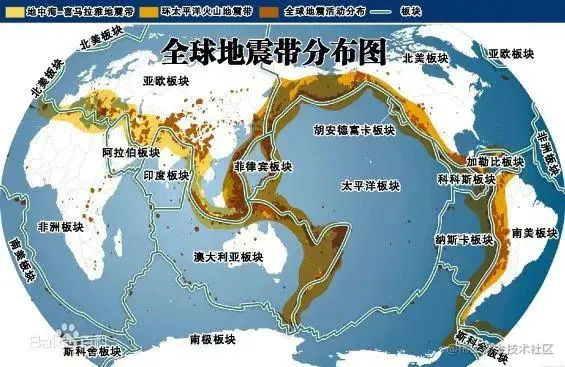We screen 5 The distribution map of earthquakes above M is as follows ：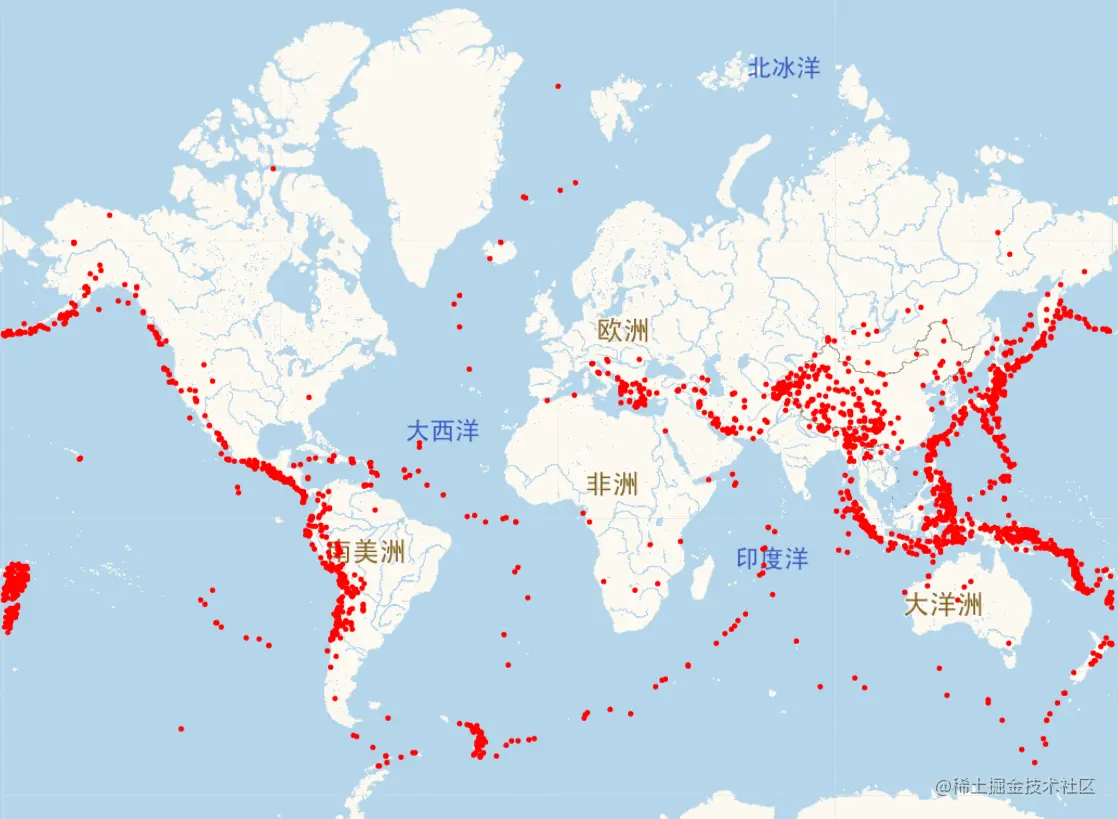Basically match ~

That's all of this , If you're interested , Order one ` Looking at ` The private chat Xiaobian can get data Ha ~